Ex 10.2

Chapter 10 Class 11 Straight Lines (Term 1)
Serial order wise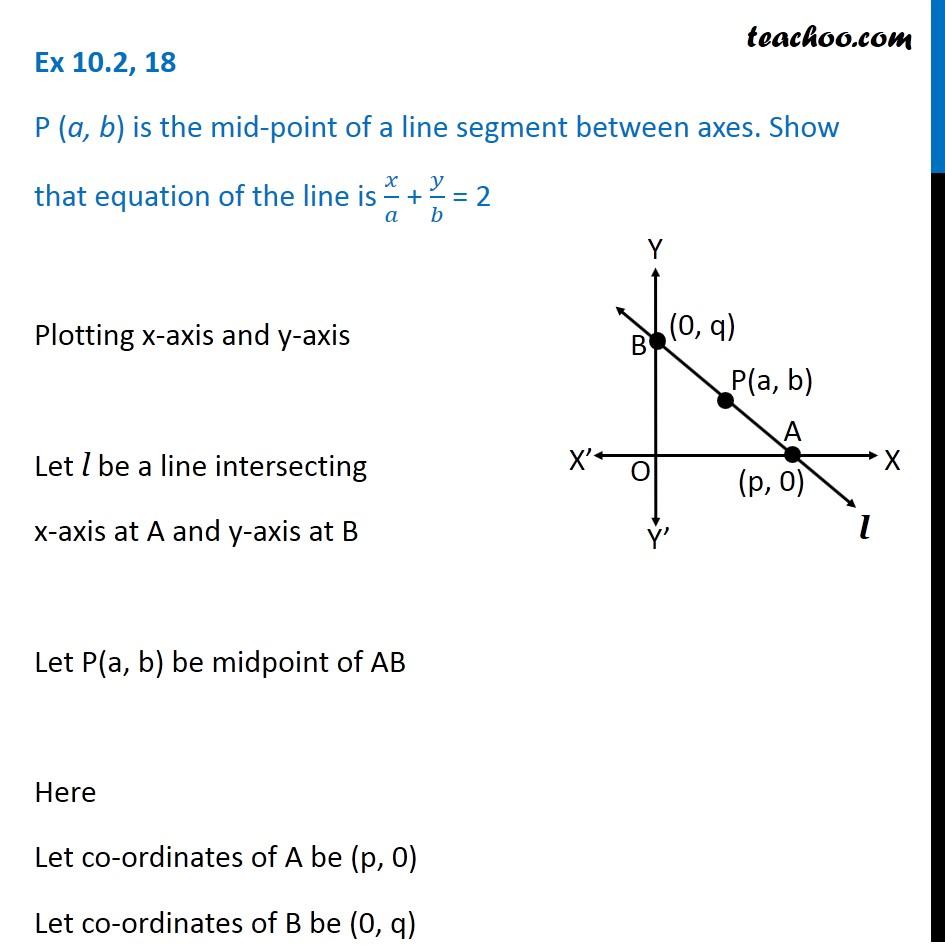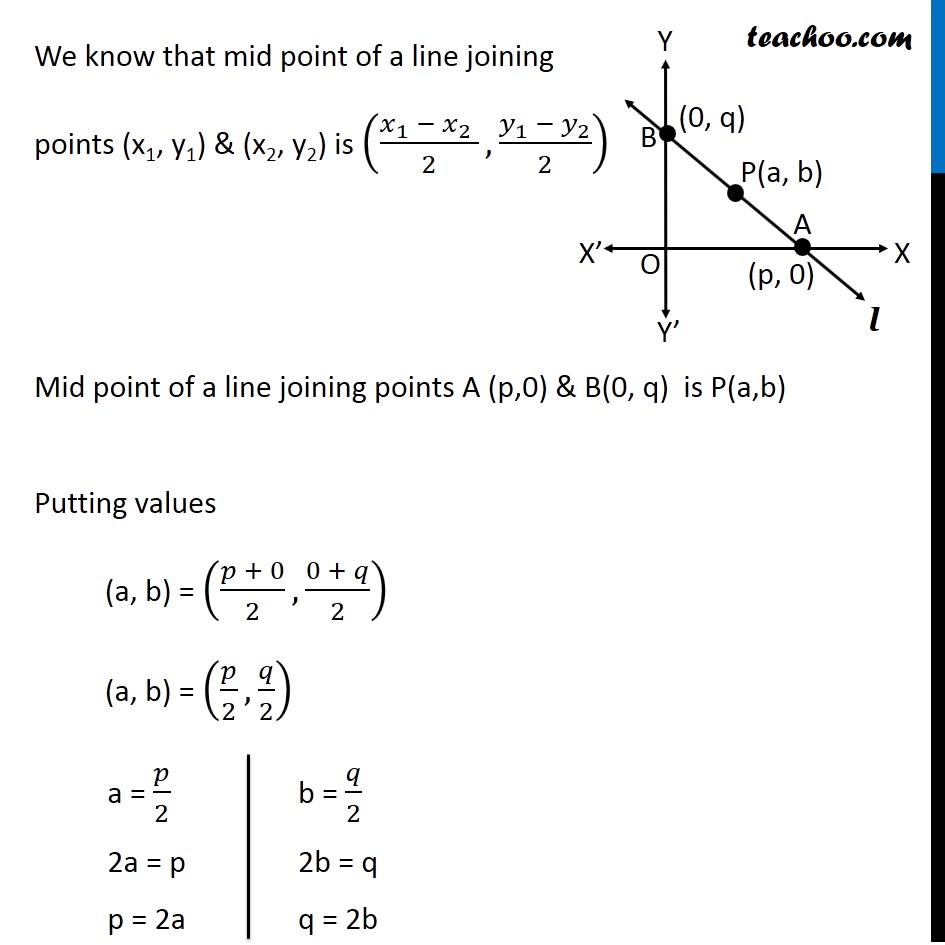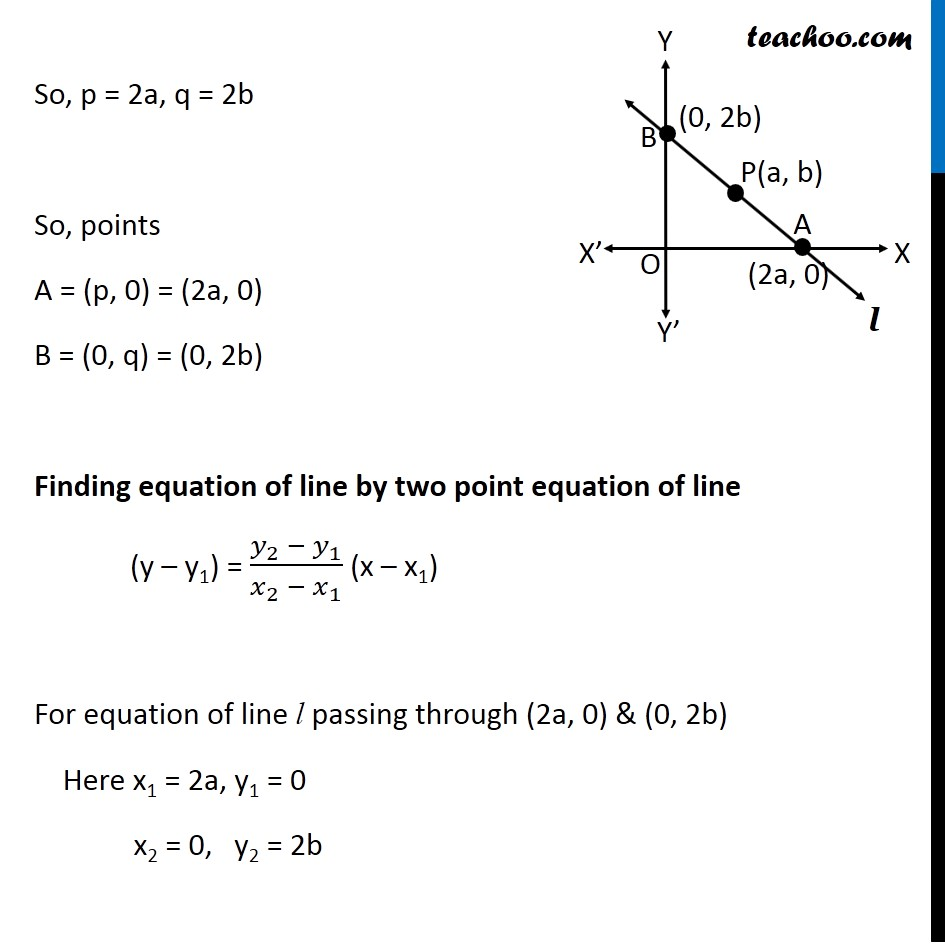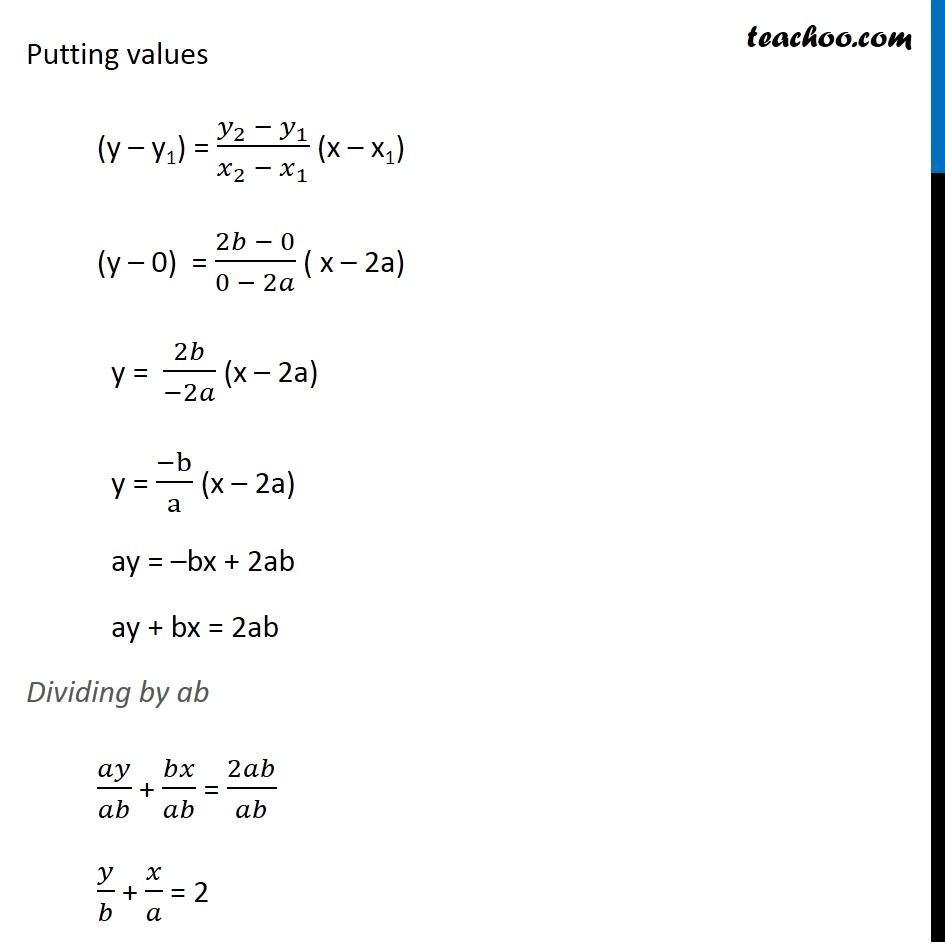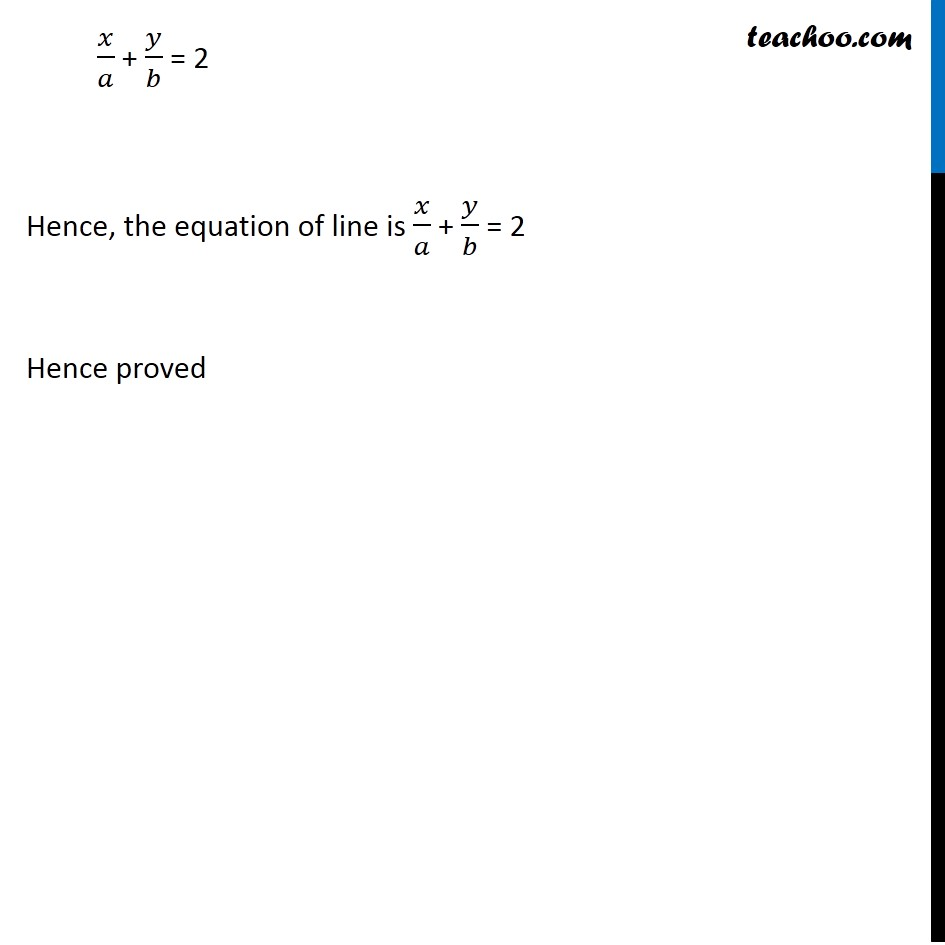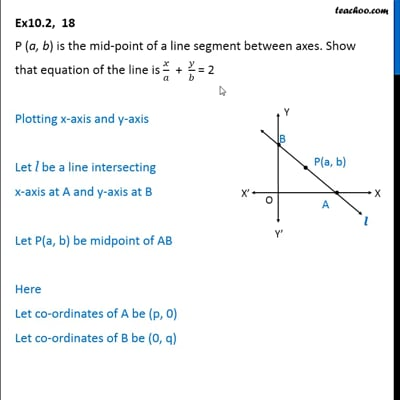This video is only available for Teachoo black users

### Transcript

Ex 10.2, 18 P (a, b) is the mid-point of a line segment between axes. Show that equation of the line is 𝑥/𝑎 + 𝑦/𝑏 = 2 Plotting x-axis and y-axis Let l be a line intersecting x-axis at A and y-axis at B Let P(a, b) be midpoint of AB Here Let co-ordinates of A be (p, 0) Let co-ordinates of B be (0, q) We know that mid point of a line joining points (x1, y1) & (x2, y2) is ((𝑥_1 − 𝑥_2 )/2, (𝑦_1 − 𝑦_2)/2) Mid point of a line joining points A (p,0) & B(0, q) is P(a,b) Putting values (a, b) = ((𝑝 + 0)/2, (0 + 𝑞)/2) (a, b) = (𝑝/2, 𝑞/2) So, p = 2a, q = 2b So, points A = (p, 0) = (2a, 0) B = (0, q) = (0, 2b) Finding equation of line by two point equation of line (y – y1) = (𝑦_2 − 𝑦_1)/(𝑥_2 − 𝑥_1 ) (x – x1) For equation of line l passing through (2a, 0) & (0, 2b) Here x1 = 2a, y1 = 0 x2 = 0, y2 = 2b Putting values (y – y1) = (𝑦_2 − 𝑦_1)/(𝑥_2 − 𝑥_1 ) (x – x1) (y – 0) = (2𝑏 − 0)/(0 − 2𝑎) ( x – 2a) y = 2𝑏/(−2𝑎) (x – 2a) y = (−b)/a (x – 2a) ay = –bx + 2ab ay + bx = 2ab Dividing by ab 𝑎𝑦/𝑎𝑏 + 𝑏𝑥/𝑎𝑏 = 2𝑎𝑏/𝑎𝑏 𝑦/𝑏 + 𝑥/𝑎 = 2 𝑥/𝑎 + 𝑦/𝑏 = 2 Hence, the equation of line is 𝑥/𝑎 + 𝑦/𝑏 = 2 Hence proved